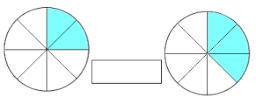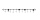What is 4 1/2+2/7-213/14?

x =  -10.4286

### Step-by-step explanation:Did you find an error or inaccuracy? Feel free to write us. Thank you!Tips to related online calculators
Need help to calculate sum, simplify or multiply fractions? Try our fraction calculator.

## Related math problems and questions:

• Fractions and mixed numerals(a) Convert the following mixed numbers to improper fractions. i. 3 5/8 ii. 7 7/6 (b) Convert the following improper fraction to mixed number. i. 13/4 ii. 78/5 (c) Simplify these fractions to their lowest terms. i. 36/42 ii. 27/45 2. evaluate following exAdd two mixed fractions: 2 4/6 + 1 3/6
• Evaluate mixed expressionsWhich of the following is equal to 4 and 2 over 3 divided by 3 and 1 over 2? A. 4 and 2 over 3 times 3 and 2 over 1 B. 14 over 3 times 2 over 7 C. 14 over 3 times 7 over 2 D. 42 over 3 times 2 over 31Add this two mixed numbers: 1 5/6 + 2 2/11=
• Between two mixedWhat is the rational number between 2 1/4 and 2 4/5?2 and 1/8th plus 1 and 1/3rd =
• Simplify 7Simplify. 7-1/3÷ 3-2/3 of 2+ 4-1/2÷ 2-1/4+ 1/2 solution and by step by stepAdd this two mixed numbers: 1 5/6 + 2 2/11=
• Difference mixed fractionsWhat is the difference between 4 2/3 and 3 1/6?
• Simplify 3Simplify mixed numerals expression: 8 1/4- 3 2/5 - (2 1/3 - 1/4) Show your solution.
• Walk for exerciseAnya, Jose, Cali, and Stephan walk for exercise. Anya's route is 2 1/4 kilometers long. Jose's route is 1 1/2 fewer km. Cali's route is 1 1/2  times as long as Jose's route, and 2 fewer km than Stephan's route. What distance (S) is Stephan's route?
• Divide 11Divide the product of 4 and 5/8 by 1 1/2. Write your answer as a mixed number.3 3/4 + 2 3/5 + 5 1/2 Show your solution.Write the equivalent multiplication expression. 2 1/6÷3/4Martin is making a model of a Native American canoe. He has 5 1/2 feet of wood. He uses 2 3/4 feet for the hull and 1 1/4 feet for a paddle. How much wood does he have left? Martin has feet of wood left.The first team member in a 926-person relay race must run 2 1/4 laps, the second team member must run 1 1/2 laps, and the third team member must run 3 1/4 laps. How many laps in all must each team run?Rewrite mixed numbers, so the fractions have the same denominator: 5 1/5 - 2 2/3